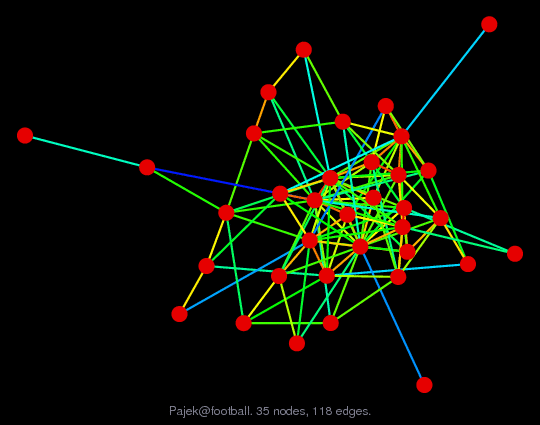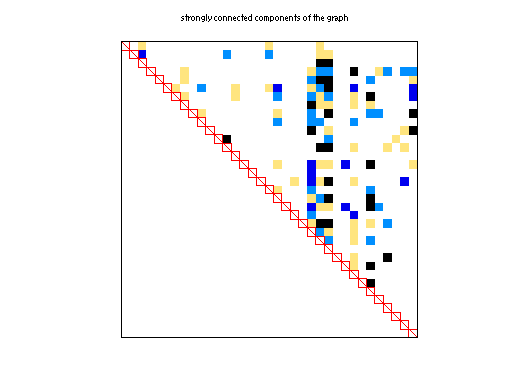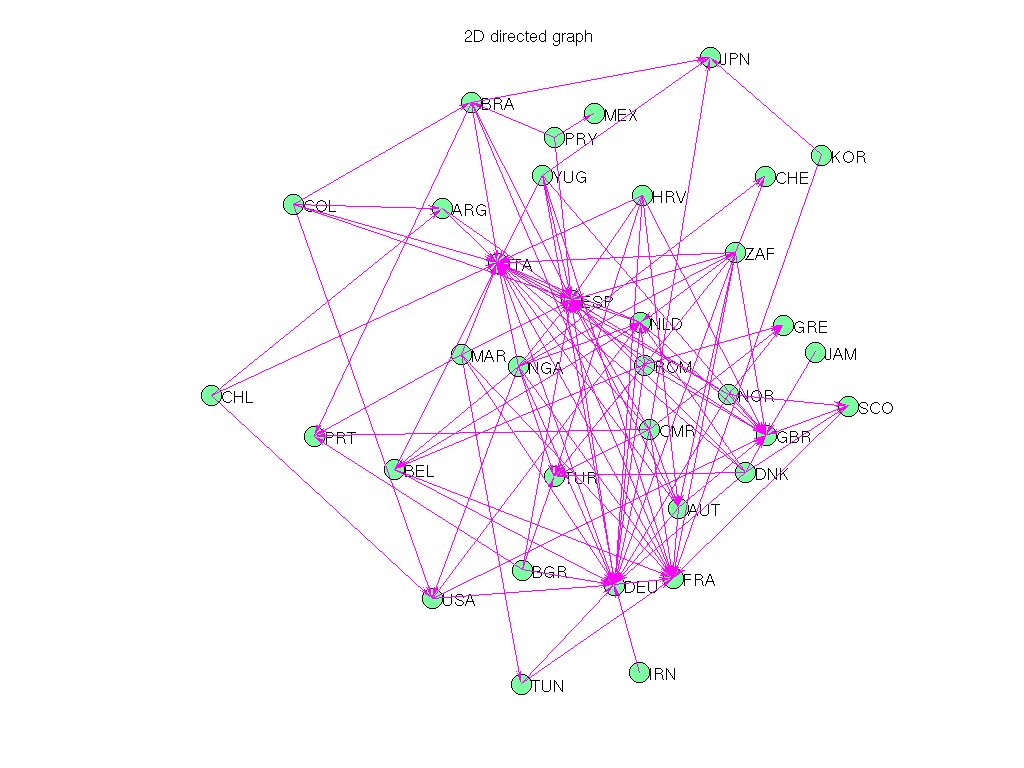Matrix: Pajek/football

Description: Pajek network: World Soccer, Paris 1998(bipartite graph drawing) (graph drawing of A+A')• Matrix group: Pajek
• download as a MATLAB mat-file, file size: 2 KB. Use UFget(1474) or UFget('Pajek/football') in MATLAB.

 Matrix properties number of rows 35 number of columns 35 nonzeros 118 # strongly connected comp. 35 explicit zero entries 0 nonzero pattern symmetry 0% numeric value symmetry 0% type integer structure unsymmetric Cholesky candidate? no positive definite? no

 author L. Krempel editor V. Batagelj date 1998 kind directed weighted graph 2D/3D problem? no

 Additional fields size and type nodename full 35-by-3 coord full 35-by-2

Notes:

```------------------------------------------------------------------------------
Pajek network converted to sparse adjacency matrix for inclusion in UF sparse
matrix collection, Tim Davis.  For Pajek datasets, See V. Batagelj & A. Mrvar,
------------------------------------------------------------------------------
The original problem had 3D xyz coordinates, but all values of z were equal
to 0.5, and have been removed.  This graph has 2D coordinates.
```

 SVD-based statistics: norm(A) 23.0386 min(svd(A)) 0 cond(A) Inf rank(A) 19 null space dimension 16 full numerical rank? no singular value gap 2.97543e+13

 singular values (MAT file): click here SVD method used: s = svd (full (A)) status: ok